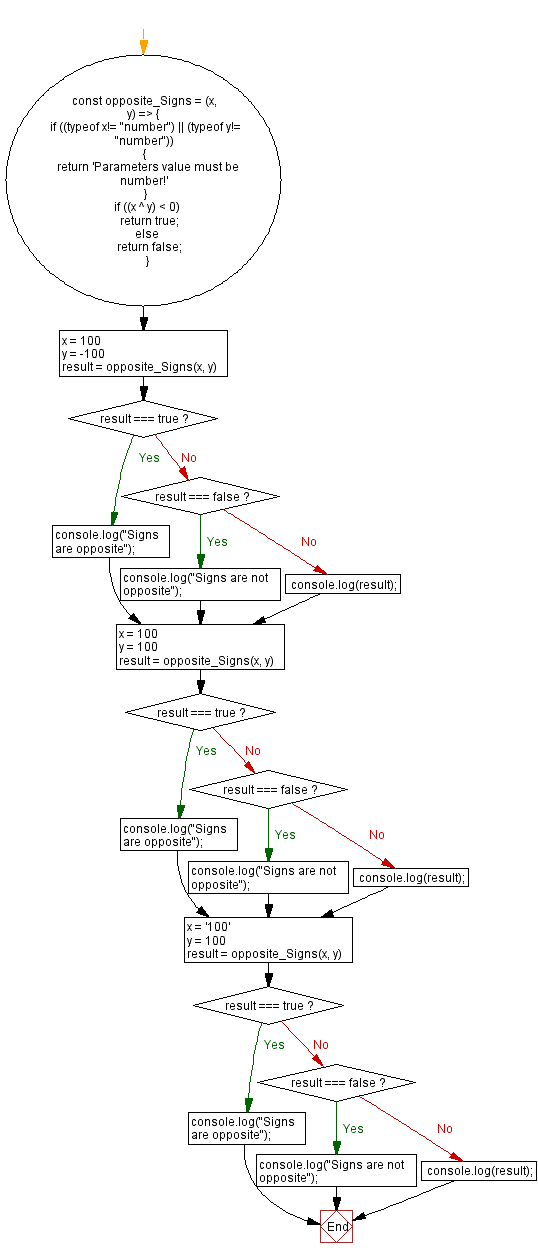# JavaScript - Check two integers have opposite signs or not

## JavaScript Bit Manipulation: Exercise-1 with Solution

Write a JavaScript program to check if two given integers have opposite signs.

Test Data:
(100, -100) -> "Signs are opposite"
(100, 100) -> "Signs are not opposite"
(‘100, 100) -> "Parameters value must be number!"

Sample Solution:

JavaScript Code:

``````const opposite_Signs = (x, y) => {
if ((typeof x!= "number") || (typeof y!= "number"))
{
return 'Parameters value must be number!'
}
if ((x ^ y) < 0)
return true;
else
return false;
}
x = 100
y = -100
result = opposite_Signs(x, y)
if (result === true)
console.log("Signs are opposite");
else if (result === false)
console.log("Signs are not opposite");
else console.log(result);
x = 100
y = 100
result = opposite_Signs(x, y)

if (result === true)
console.log("Signs are opposite");
else if (result === false)
console.log("Signs are not opposite");
else console.log(result);
x = '100'
y = 100
result = opposite_Signs(x, y)
if (result === true)
console.log("Signs are opposite");
else if (result === false)
console.log("Signs are not opposite");
else console.log(result);
```
```

Sample Output:

```Signs are opposite
Signs are not opposite
Parameters value must be number!
```

Flowchart:Live Demo:

See the Pen javascript-date-exercise-54 by w3resource (@w3resource) on CodePen.

* To run the code mouse over on Result panel and click on 'RERUN' button.*

Improve this sample solution and post your code through Disqus

What is the difficulty level of this exercise?

Test your Programming skills with w3resource's quiz.

﻿